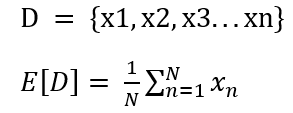#2019 iT 邦幫忙鐵人賽DAY 3
0

## Day 3-機器學習與數學天天玩-PCA-Statistical Introduction: Mean Value

Today is Staturday and I will continue my study on Coursera course with a simple summary.

Like what I shared yesterday, the brief structure leading to the milestone of PCA is as below:
1. Statistical Introduction
2. Transformation of Vectors in Spaces
3. Orthogonal Projection

In the statistical introduction, the course firstly introduces the meaning of mean value in a dataset D. The mean value is to have the summation of all elements in a dataset divided by the total number of elements.Along with the course, a useful function marked as one of fundamental tools in develping PCA skill is: flatten()

``````x = np.matrix([[1, 2], [3, 4]])
``````

Out:
matrix([[1, 2],
[3, 4]])

``````x_reshaped = x.flatten()
``````

Out: matrix([[1, 2, 3, 4]])

By observing the above code, it is apparent that flatten() helps tranform values in a matrix from several layers into one row.